Function Repository Resource:

# InactiveRiemannSumToIntegral

Determine an integral corresponding to a Riemann sum

Contributed by: Wolfram|Alpha Math Team
 ResourceFunction["InactiveRiemannSumToIntegral"][fun,{i,1,n},n→Infinity,x] expresses the Riemann sum of fun over i from 1 to n as an integral in the variable x.

## Examples

### Basic Examples (1)

Find the integral corresponding to the Riemann sum with summand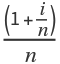:

 In:=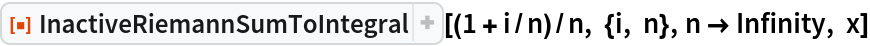Out=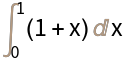### Scope (2)

Find the integral corresponding to the Riemann sum with summand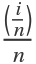:

 In:=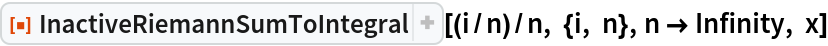Out=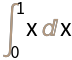Find the integral corresponding to the Riemann sum with summand Cos[(1+i)/n]/n:

 In:=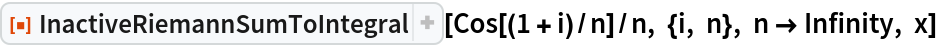Out=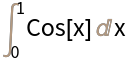### Possible Issues (1)

If an integral could not be found, an inactivated form of the limit is returned with a message:

 In:=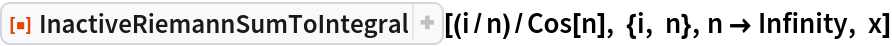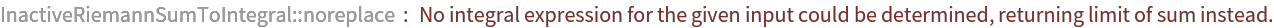Out=## Publisher

Wolfram|Alpha Math Team

## Version History

• 3.0.0 – 23 March 2023
• 2.0.0 – 08 October 2021

## Author Notes

To view the full source code for InactiveRiemannSumToIntegral, evaluate the following:

 In:=